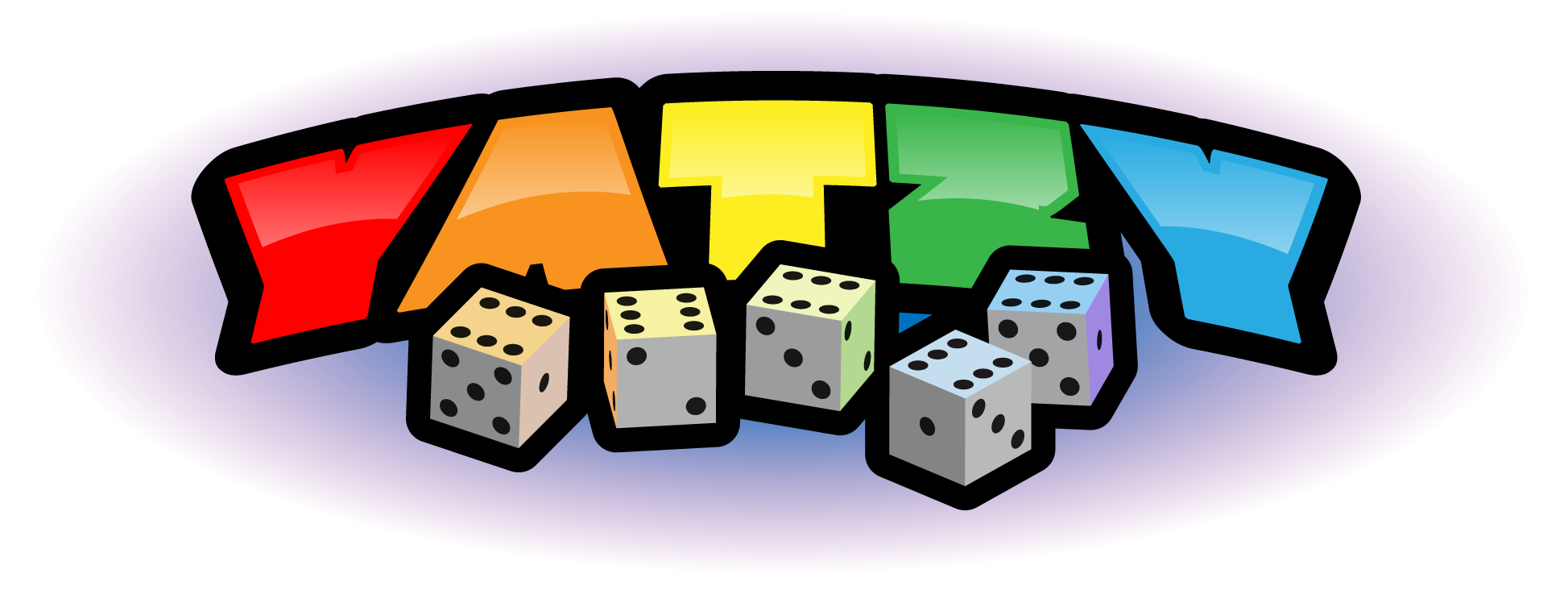Press the + button in the top right corner to install this app.

# How to Play

Yatzy can be played solitaire or by any number players. Players take turns rolling five dice. After each roll, the player chooses which dice to keep, and which to reroll. A player may reroll some or all of the dice up to two times on a turn. The player with the highest total score wins the game. After each round (3 rolls / round) points must be entered into a table.

## Scoring system

The following combinations earn points:

Upper Section:

• Ones: The sum of all dice showing the number 1.
• Twos: The sum of all dice showing the number 2.
• Threes: The sum of all dice showing the number 3.
• Fours: The sum of all dice showing the number 4.
• Fives: The sum of all dice showing the number 5.
• Sixes: The sum of all dice showing the number 6.

Lower Section:

• One Pair: Two dice showing the same number. Score: Sum of those two dice.
• Two Pairs: Two pairs of dice. Score: Sum of dice in those two pairs.
• Three of a Kind: Three dice showing the same number. Score: Sum of those three dice.
• Four of a Kind: Four dice with the same number. Score: Sum of those four dice.
• Small Straight: The combination 1, 2, 3, 4, 5. Score: 15 points (sum of all the dice).
• Large Straight: The combination 2, 3, 4, 5, 6. Score: 20 points (sum of all the dice).
• House: Any set of three combined with a set of two. Score: Sum of all the dice.
• Yatzy: All five dice with the same number. Score: 50 points.
• Chance: Any combination of dice. Score: Sum of all the dice.
• Bonus: If a player manages to score at least 63 points (three of each number) in the upper section, they are awarded a bonus of 50 points (in some instances 25 or 35).

## Other good things to know

• You should generally aim for the 35 points bonus by filling the upper section with high scores (so as to get at least 63 points) near the beginning of the game.
• If you roll a combination for which your available boxes give a low score, fill in a zero in the 1's box (or even the 2's or 3's), since high scores in the other boxes may compensate for this.
• Early in the game you should use the chance box only as a fall-back for when you are trying for a high point combination and fail. You want to keep the chance box available for a situation where your options are very limited.
• If you have a bad roll of the dice near the end of the game, you may enter a zero in the Yahtzee box or one of the other difficult combinations.
• If you roll a pair of 1's or 2's early in the game, go for a full house, straight, or one of the other high numbers you rolled. Keeping the 1's or 2's to seek a 3 or 4 of a kind will not get you many points. Plus, it is a good idea to keep the 1's and 2's boxes available for later in the game to have the possibility of putting a zero there. As an example, if on your first turn your initial roll is 1 1 2 5 6, it turns out that keeping only the 5 is the choice that maximizes your expected score for the game.
• If you roll four of a kind with 4's, 5's, or 6's, take the points in the upper section if they are available, not in the 4 of a kind box. This will help you get the 35 points bonus at the end of the game.
• You should always try for a Yahtzee early in the game. If you roll a 3 or 4 of a kind on your first or second roll and you have the corresponding box in the upper section open, you should definitely go for the Yahtzee. Plus, if you saved your chance, 1's, or 2's boxes, you can score a zero there if you fail to get the Yahtzee."
Yatzy on Wikipedia

## Developers

This application has been developed by iMind and Euedge and it was designed by trn.

# Game over

## Total score:

 Upper score: +bonus! Total score: